back to main page

Examples of use of n!

Combinatorics

The binomial coefficient of the natural number n and the integer k is defined to be the natural number: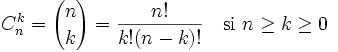and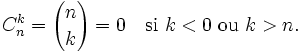Partial derivative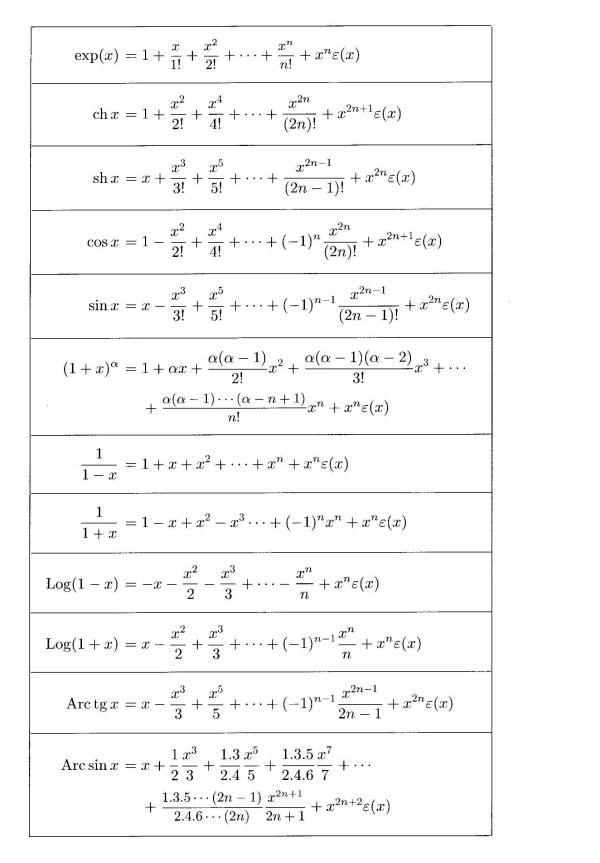Stirling's formula

Asymptotic series expansions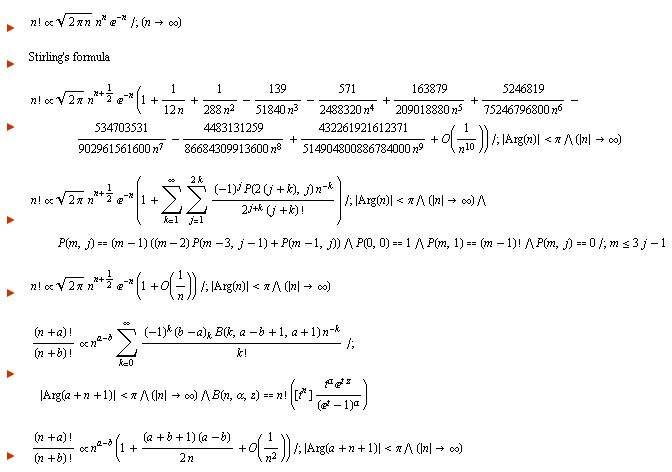Function with n!

Gamma function.
If the real part of the complex number z is positive, then the following integral converges absolutely: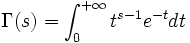.
In particular, using integration by parts, we can show that: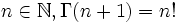.

Stirling's approximation:
http://mathworld.wolfram.com/StirlingsApproximation.html

Factorial and permutations:
http://mathworld.wolfram.com/Factorial.html

96 other formulas with this notation:
http://functions.wolfram.com/GammaBetaErf/Factorial/

Gamma and Beta functions:
http://functions.wolfram.com/GammaBetaErf/

back to main page